Processing ......FreeComputerBooks.com Links to Free Computer, Mathematics, Technical Books all over the World

Calculus and Mathematical Analysis (Real Analysis, Functional Analysis, etc.)
Related Book Categories:
•Calculus (Gilbert Strang)

This highly regarded calculus textbook is ideal as for self-study. Examples of apps of calculus to physics, engineering and economics are included, as well as many practice questions and illustrative diagrams to assist the reader's grasp of the material.

•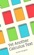Yet Another Calculus Text: A Short Introduction with Infinitesimals

An introduction to calculus based on the hyperreal number system so it uses infinitesimal and infinite numbers freely. It aims primarily at readers who already have some familiarity with calculus.

•Measure, Integration and Real Analysis (Sheldon Axler)

This textbook welcomes students into the fundamental theory of measure, integration, and real analysis. Focusing on an accessible approach, it lays the foundations for further study by promoting a deep understanding of key results.

•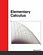Elementary Calculus (Michael Corral)

This textbook covers calculus of a single variable, is designed for students who have completed courses in high-school algebra, geometry, and trigonometry. Though designed for college students, it could also be used in high schools.

•Calculus Made Easy (Silvanus P. Thompson)

This book is a popular math book to help modern readers of all levels understand the subject on calculus through simple way and explanation. It's not just theory-based but contains exercises and answers so you can practice the understanding for each chapter.

•Precalculus: An Investigation of Functions (David Lippman, et al)

Comprehensive and evenly paced, this book provides complete coverage of the function concept, and integrates a significant amount of graphing calculator material to help students develop insight into mathematical ideas.

•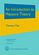An Introduction to Measure Theory (Terrence Tao)

This is a graduate text introducing the fundamentals of measure theory and integration theory, which is the foundation of modern real analysis, intended to cover a quarter or semester's worth of material for a first graduate course in real analysis.

•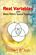Real Variables with Basic Metric Space Topology (Robert B. Ash)

Designed for a first course in real variables, this text presents the fundamentals for more advanced mathematical work, particularly in the areas of complex variables, measure theory, differential equations, functional analysis, and probability.

•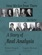How We Got from There to Here: A Story of Real Analysis

This book is an introductory real analysis textbook, presented through the lens of history. The definitions and techniques are motivated by the actual difficulties encountered by the intuitive approach and are presented in their historical context.

•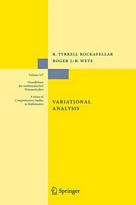Variational Analysis (R. Tyrrell Rockafellar, et al)

This book develops a unified framework and, in finite dimension, provides a detailed exposition of variational geometry and subdifferential calculus in their current forms beyond classical and convex analysis.

•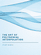The Art of Polynomial Interpolation (Stuart Murphy)

Exploring the techniques of interpolating data allows us to view the development and birth of a polynomial. This book is focused on laying a foundation for understanding and applying several common forms of Polynomial Interpolation.

•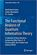The Functional Analysis of Quantum Information Theory

This book provides readers with a concise introduction to current studies on operator-algebras and their generalizations, operator spaces and operator systems, with a special focus on their application in Quantum Information Science.

•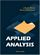Applied Analysis (John K. Hunter, et al)

This book provides an introduction to those parts of analysis that are most useful in applications for graduate students. The material is selected for use in applied problems, and is presented clearly and simply but without sacrificing mathematical rigor.

•Calculus: Theory and Applications (Kenneth Kuttler)

This is a book on single variable calculus including most of the important applications of calculus. It also includes proofs of all theorems presented, either in the text itself, or in an appendix. It also contains an introduction to vectors and vector products.

•Advanced Calculus (Lynn H. Loomis, et al)

Problem solving and mathematical modeling are introduced early and reinforced throughout, providing students with a solid foundation in the principles of mathematical thinking.

•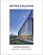Active Calculus (Matt Boelkins)

Rather than detailed explanations and worked out examples, this book uses activities intended to be done by the students in order to present the standard concepts and computational techniques of calculus.

•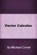Vector Calculus (Michael Corral)

This is a text on elementary multivariable calculus, designed for students who have completed courses in single-variable calculus. Concepts are amply illustrated with figures, worked examples, and physical applications.

•Calculus (WikiBooks)

This wikibook aims to be a high quality calculus textbook through which users can master the discipline. Standard topics such as limits, differentiation and integration are covered, as well as several others.

•Introduction to Mathematical Analysis (Beatriz Lafferriere, et al)

The goal of this book is to provide students with a strong foundation in mathematical analysis. Such a foundation is crucial for future study of deeper topics of analysis. It will be reinforced through rigorous proofs.

•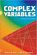Complex Variables: Second Edition (Robert B. Ash, et al)

This text on complex variables is geared toward who have taken an introductory course in real analysis. Offering a concise treatment that provides careful and complete explanations as well as numerous problems and solutions.

•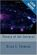Theory of the Integral (Brian S. Thomson)

This text is intended as a treatise for a rigorous course introducing the elements of integration theory on the real line. All of the important features of the Riemann integral, the Lebesgue integral, and the Henstock-Kurzweil integral are covered.

•Complex Integration and Cauchy's Theorem (G.N. Watson)

This brief monograph by one of the great mathematicians offers a single-volume compilation of propositions employed in proofs of Cauchy's theorem, provides a brief account of the various applications of the theorem to the evaluation of definite integrals.

•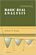Basic Real Analysis (Anthony W. Knapp)

This is a comprehensive treatment with a global view of the Real Analysis, emphasizing the connections between real analysis and other branches of mathematics. Included throughout are many examples and hundreds of problems.

•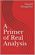A Primer of Real Analysis (Dan Sloughter)

This is a short introduction to the fundamentals of real analysis, written the text assuming the reader has the level of mathematical maturity of one who has completed the standard sequence of calculus courses.

•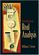Introduction to Real Analysis (William F. Trench)

Using an extremely clear and informal approach, this book introduces readers to a rigorous understanding of mathematical analysis and presents challenging math concepts as clearly as possible.

•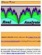Interactive Real Analysis (Bert G. Wachsmuth)

An interactive textbook for Real Analysis or Advanced Calculus in one real variable. It deals with sets, sequences, series, continuity, differentiability, integrability (Riemann and Lebesgue), topology, power series, and more.

•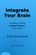Integrate Your Brain: How To Do Calculus In Your Head

This book teaches how talented mathematicians utilize their normal, human mental ability to tackle calculus. You are gently trained in the fundamental skills, and shown step by step how to put them into action yourself.

•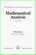Mathematical Analysis I (Elias Zakon)

This book carefully leads the student through the basic topics of real analysis. Topics include metric spaces, open and closed sets, convergent sequences, function limits and continuity, compact sets, sequences and series of functions, power series, etc.

•Mathematical Analysis II (Elias Zakon)

It is for any second course in real analysis or mathematical analysis, covering Banach spaces, measure theory (with a complete discussion of outer measures, Lebesgue measure, Lebesgue-Stieltjes measures, and differentiation of set functions), etc.

•Elementary Calculus: An Infinitesimal Approach (H. Jerome Keisler)

This first-year calculus book is centered around the use of infinitesimals. It contains all the ordinary calculus topics, including the basic concepts of the derivative, continuity, and the integral, plus traditional limit concepts and approximation problems.

•Foundations of Infinitesimal Calculus (H. Jerome Keisler)

This monograph is a companion to the Author's textbook. It can be used as a quick introduction to the infinitesimal approach to calculus for mathematicians, as background material for instructors, or as a text for an undergraduate seminar.

•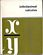Mathematical Background: Foundations of Infinitesimal

We wrote this book of foundations in part to provide a convenient reference for a student who might like to see the "theorem - proof" approach to calculus. It also shows how the pathologies arise in the traditional approach.

•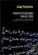Nonstandard Analysish (Jaap Ponstein, et al.)

This book is concerned with an attempt to introduce the infinitesimals and the other 'nonstandard' numbers in a naive, simpleminded way. Nevertheless, the resulting theory is hoped to be mathematically sound, and to be complete within obvious limits.

•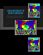Linear Mathematics In Infinite Dimensions (Ulrich H. Gerlach)

Focus on the mathematical framework that underlies linear systems arising in physics, engineering and applied mathematics - from the theory of linear transformation on finite dimensional vector space to the infinite dimensional vector spaces.

•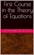First Course in the Theory of Equations (Leonard Dickson)

The theory of equations is not only a necessity in the subsequent mathematical courses and their applications, but furnishes an illuminating sequel to geometry, algebra and analytic geometry. A useful supplement to differential calculus.

•Analysis and Linear Algebra for Finance (Patrick Roger)

This book present the elements of analysis and linear algebra used in financial models and in microeconomics. It provides a broad overview of the mathematics of financial models. Functions of one and several variables and matrices are developed.

Book Categories
 :All CategoriesRecent BooksMiscellaneous BooksComputer LanguagesComputer ScienceData Science/DatabasesElectrical EngineeringJava and Java EE (J2EE)Linux and UnixMathematicsMicrosoft and .NETMobile ComputingNetworking and CommunicationsSoftware EngineeringSpecial TopicsWeb Programming
Other Categories